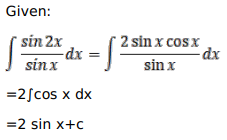# Mark against the correct answer in each of the following:

Question:

Mark $(\sqrt{)}$ against the correct answer in each of the following:

$\int \frac{\sin 2 x}{\sin x} d x=?$

A. $2 \sin x+C$

B. $\frac{1}{2} \sin x+C$

C. $2 \cos x+C$

D. $\frac{1}{2} \cos x+C$

Solution: